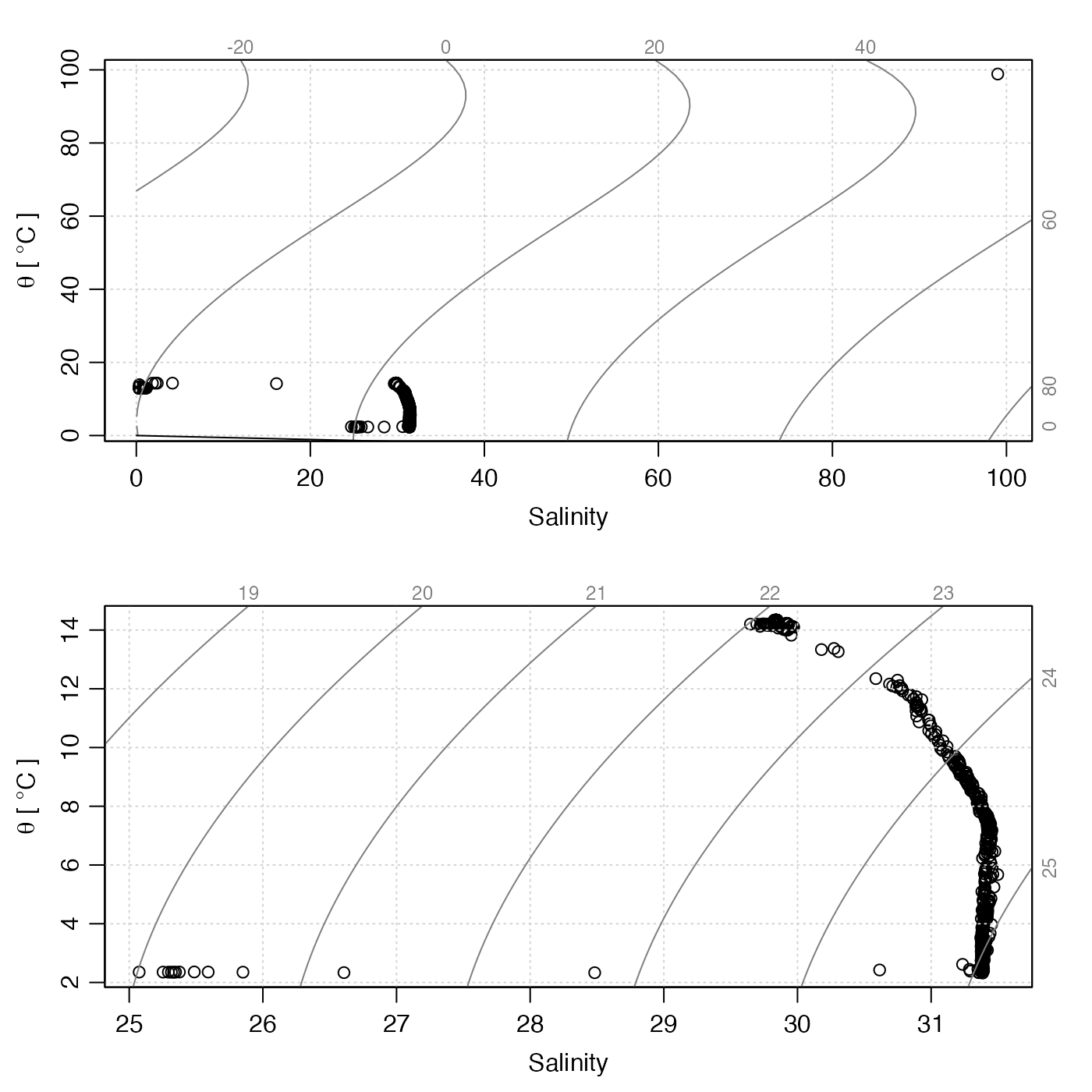This function changes specified entries in the data-quality flags of a ctd object, which are stored within a list named flags that resides in the metadata slot. If the object already has a flag set up for name, then only the specified entries are altered. If not, the flag entry is first created and its entries set to default, after which the entries specified by i are changed to value.

The specification is made with i, the form of which is determined by the data item in question. Generally, the rules are as follows:

1. If the data item is a vector, then i must be (a) an integer vector specifying indices to be set to value, (b) a logical vector of length matching the data item, with TRUE meaning to set the flag to value, or (c) a function that takes an oce object as its single argument, and returns a vector in either of the forms just described.

2. If the data item is an array, then i must be (a) a data frame of integers whose rows specify spots to change (where the number of columns matches the number of dimensions of the data item), (b) a logical array that has dimension equal to that of the data item, or (c) a function that takes an oce object as its single input and returns such a data frame or array.

See “Details” for the particular case of ctd objects.

# S4 method for ctd
setFlags(
object,
name = NULL,
i = NULL,
value = NULL,
debug = getOption("oceDebug")
)

## Arguments

object

An oce object.

name

Character string indicating the name of the variable to be flagged. If this variable is not contained in the object's data slot, an error is reported.

i

Indication of where to insert the flags; see “Description” for general rules and “Details” for rules for ctd objects.

value

The value to be inserted in the flag.

debug

Integer set to 0 for quiet action or to 1 for some debugging.

## Value

An object with flags set as indicated.

## Details

Since all the entries in the data slot of ctd objects are vectors, i must be a vector (either logical as in Example 1 or integer as in Example 2), or a function taking a ctd object and returning such a vector (see “Indexing rules”).

Other functions relating to data-quality flags: defaultFlags(), handleFlags,adp-method, handleFlags,argo-method, handleFlags,ctd-method, handleFlags,oce-method, handleFlags,section-method, handleFlags(), initializeFlagScheme,ctd-method, initializeFlagScheme,oce-method, initializeFlagScheme,section-method, initializeFlagSchemeInternal(), initializeFlagScheme(), initializeFlags,adp-method, initializeFlags,oce-method, initializeFlagsInternal(), initializeFlags(), setFlags,adp-method, setFlags,oce-method, setFlags()

Other things related to ctd data: CTD_BCD2014666_008_1_DN.ODF.gz, [[,ctd-method, [[<-,ctd-method, as.ctd(), cnvName2oceName(), ctd-class, ctd.cnv, ctdDecimate(), ctdFindProfiles(), ctdRaw, ctdRepair(), ctdTrim(), ctd_aml.csv, ctd, d200321-001.ctd, d201211_0011.cnv, handleFlags,ctd-method, initialize,ctd-method, initializeFlagScheme,ctd-method, oceNames2whpNames(), oceUnits2whpUnits(), plot,ctd-method, plotProfile(), plotScan(), plotTS(), read.ctd.aml(), read.ctd.itp(), read.ctd.odf(), read.ctd.odv(), read.ctd.sbe(), read.ctd.ssda(), read.ctd.woce.other(), read.ctd.woce(), read.ctd(), subset,ctd-method, summary,ctd-method, woceNames2oceNames(), woceUnit2oceUnit(), write.ctd()

## Examples

library(oce)
# Example 1: Range-check salinity
data(ctdRaw)
# Salinity and temperature range checks
qc <- ctdRaw
# Initialize flags to 2, meaning good data in the default
# scheme for handleFlags(ctd).
qc <- initializeFlags(qc, "salinity", 2)
qc <- initializeFlags(qc, "temperature", 2)
# Flag bad salinities as 4
oddS <- with(qc[["data"]], salinity < 25 | 40 < salinity)
qc <- setFlags(qc, name="salinity", i=oddS, value=4)
# Flag bad temperatures as 4
oddT <- with(qc[["data"]], temperature < -2 | 40 < temperature)
qc <- setFlags(qc, name="temperature", i=oddT, value=4)
# Compare results in TS space
par(mfrow=c(2, 1))
plotTS(ctdRaw)
plotTS(handleFlags(qc, flags=c(1, 3:9)))# Example 2: Interactive flag assignment based on TS plot, using
# WHP scheme to define 'acceptable' and 'bad' codes
if (FALSE) {
options(eos="gsw")
data(ctd)
qc <- ctd
qc <- initializeFlagScheme(qc, "WHP CTD")
qc <- initializeFlags(qc, "salinity", 2)
Sspan <- diff(range(qc[["SA"]]))
Tspan <- diff(range(qc[["CT"]]))
n <- length(qc[["SA"]])
par(mfrow=c(1, 1))
plotTS(qc, type="o")
message("Click on bad points; quit by clicking to right of plot")
for (i in seq_len(n)) {
xy <- locator(1)
if (xy$x > par("usr")) break i <- which.min(abs(qc[["SA"]] - xy$x)/Sspan + abs(qc[["CT"]] - xy\$y)/Tspan)
qc <- setFlags(qc, "salinity", i=i, value=4)
qc <- handleFlags(qc, flags=list(salinity=4))
plotTS(qc, type="o")
}
}